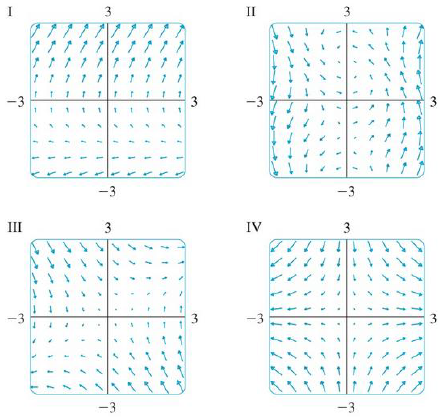Chapter 16.1, Problem 11E

Chapter
Section
Textbook Problem

Match the vector fields F with the plots labeled I-IV. Give reasons for your choices.11. F(x, y) = ⟨x, −y⟩To determine

To match: The vector field F(x,y)=x,y with the plots labeled as I-IV.

Explanation

Given data:

The vector field F(x,y)=x,y

Formula used:

The expression for the length of the two-dimensional vector F=x,y is,

|F(x,y)|=x2+y2 (1)

Calculation:

Find the length of F(x,y) using equation (1).

|F(x,y)|=(x)2+(y)2=x2+y2

Consider a certain interval of x as (2,2) and y as (2,2) to plot F(x,y).

The estimated values of |F(x,y)| and F(x,y) for different values of x and y are shown in Table 1.

Table 1

 Quadrant (x,y) |F(x,y)|=x2+y2 F(x,y)=〈x,−y〉 I (0,0) 0 〈0,0〉 (1,0) 1 〈1,0〉 (2,0) 2 〈2,0〉 (0,1) 1 〈0,−1〉 (1,1) 2 〈1,−1〉 (0,2) 2 〈0,

Still sussing out bartleby?

Check out a sample textbook solution.

See a sample solution

The Solution to Your Study Problems

Bartleby provides explanations to thousands of textbook problems written by our experts, many with advanced degrees!

Get Started

Convert the expressions in Exercises 8596 radical form. (x1/2y1/3)1/5

Finite Mathematics and Applied Calculus (MindTap Course List)

In Exercises 2340, find the indicated limit. 30. limx0(4x520x2+2x+1)

Applied Calculus for the Managerial, Life, and Social Sciences: A Brief Approach

For y = sin2 x + cos2 x, y = _____. a) 2 sin x 2 cos x b) 2 sin x cos x c) 4 sin x cos x d) 0

Study Guide for Stewart's Single Variable Calculus: Early Transcendentals, 8th